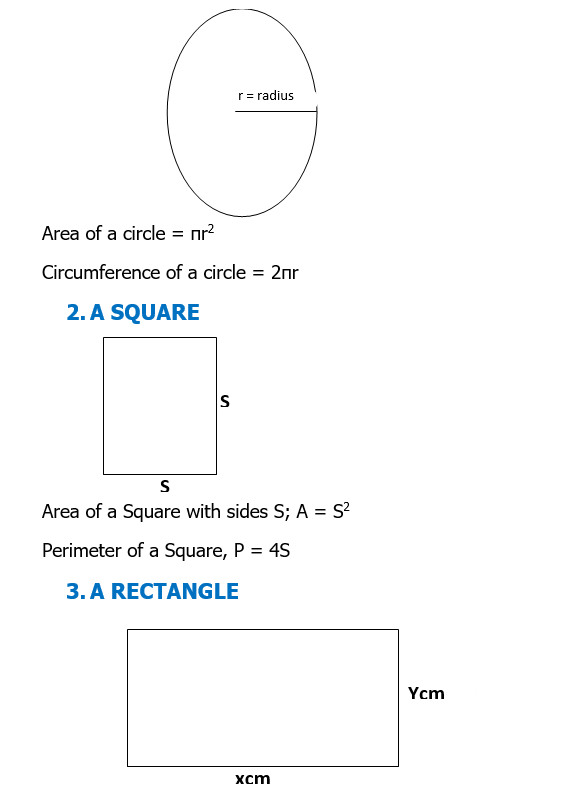### Similar Shapes 2

Mathematics J.S.S 3 Second Term

Theme: Algebraic Process

Sub Theme: Algebraic Operation

WEEK 8

Similar Shapes 2

Performance Objectives

Students should be able to;

1. Calculate length and area of similar figures
2. Calculate volumes of similar figures
3. Solve problems on quantitative reasoning involving similar shapes.

Length, Area and Volume of Similar Figure

Content

Areas of Plane Shape

1.  A CIRCLE

The formulae for calculating the area and circumference of a circle are;Area of a rectangle of length xcm  and width ycm is; A = XY

Perimeter.... View More